#Function Repository Resource:

# Monge

Return the Monge point and six midplanes of a tetrahedron

Contributed by: Ed Pegg Jr
 ResourceFunction["Monge"][vertices] returns the Monge point and six midplanes of the tetrahedron defined by vertices.

## Details and Options

In a tetrahedron, a midplane is perpendicular to one edge and concurrent with the midpoint of the opposing edge. Each tetrahedron has six midplanes, which intersect at the Monge point.

## Examples

### Basic Examples

Find the Monge point and six midplanes for a tetrahedron:

 In:=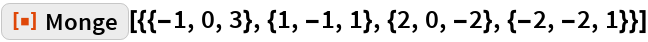Out=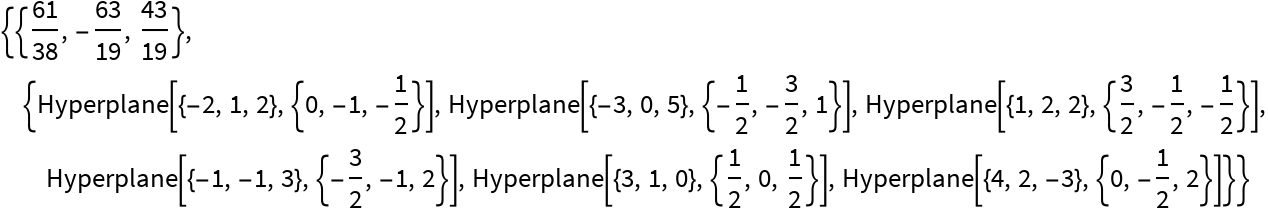A graphic of a tetrahedron, the Monge point and the six midplanes:

 In:=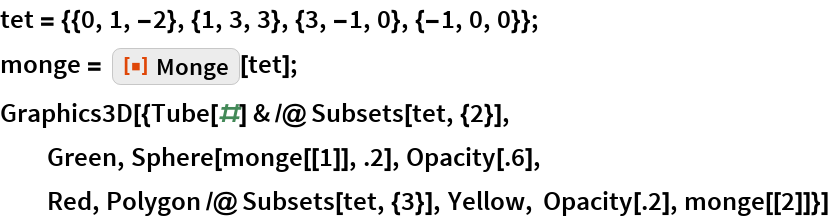Out=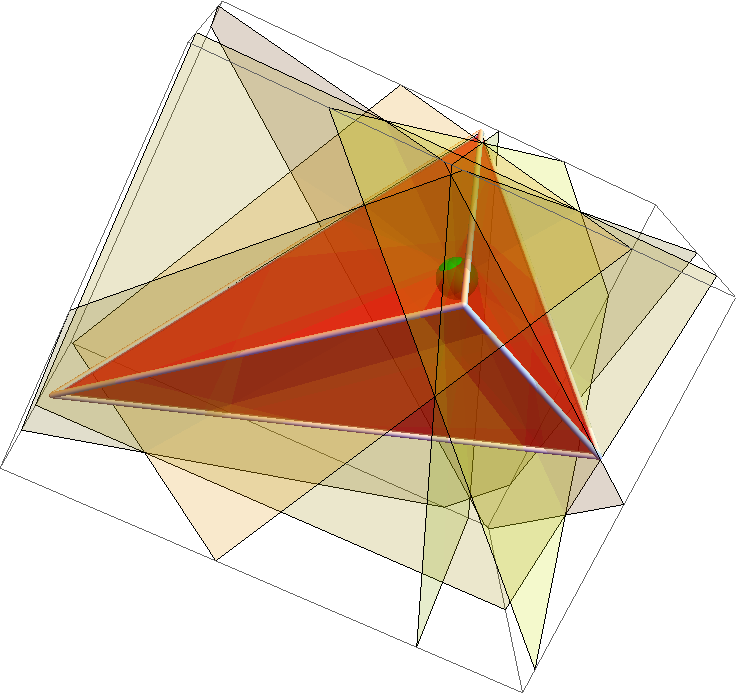### Scope

The Monge point can be outside of the tetrahedron:

 In:=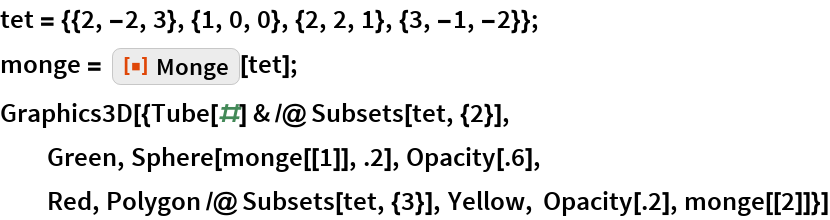Out=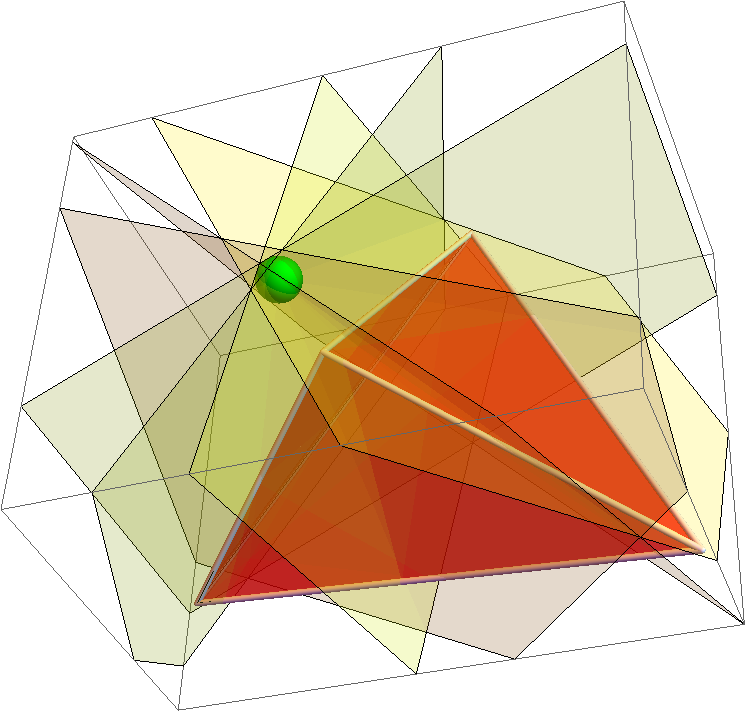## Requirements

Wolfram Language 11.3 (March 2018) or above